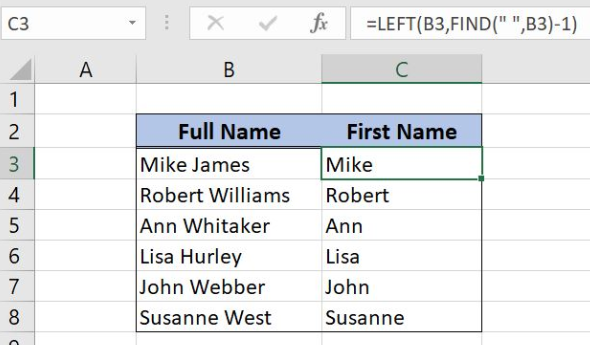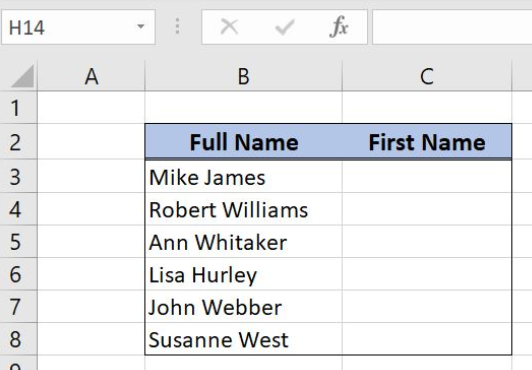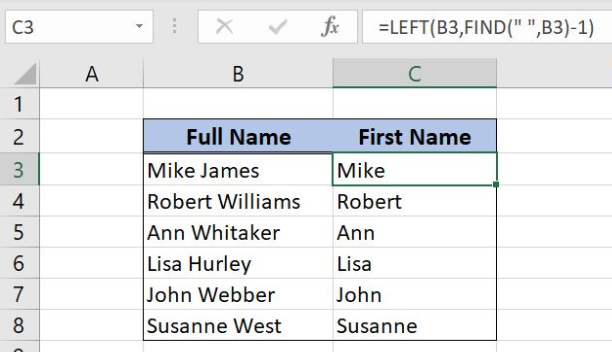Get instant live expert help with Excel or Google Sheets“My Excelchat expert helped me in less than 20 minutes, saving me what would have been 5 hours of work!”

#### Post your problem and you’ll get Expert help in seconds.

Your message must be at least 40 characters
Our professional Expert are available now. Your privacy is guaranteed.

# Get first name from name

Excel allows us to get first name from name using the FIND and LEFT functions. This step by step tutorial will assist all levels of Excel users in getting first name from nameFigure 1. The final result of the formula

## Syntax of the FIND Formula

The generic formula for the FIND function is:

`=FIND(find_text, within_text)`

The parameters of the FIND function are:

• find_text – a text that we want to find in selected text or cell
• within_text – a text or cell where we want to find a text.

If a text is found, the FIND function returns a position of text in a cell. If it’s not found, the function returns #VALUE! error.

## Syntax of the LEFT Formula

The generic formula for the LEFT function is:

`=LEFT(text, [num_chars])`

The parameters of the LEFT function are:

• text – a text from which we want to get a first part
• [num_chars] – a number of characters that we want to get from a text from the left side. This is the optional parameter, but if it is omitted, the function will return the text itself.

## Setting up Our Data for the Example

Our table consists of w columns: “Full Name” (column B) and “First Name” (column C). In column C, we want to get the first name from a full name.Figure 2. Data that we will use in the examples

## Get the First Name from the Name

In the example, we want to get the first name in the cell C3, from the full name in B3. We will first find a space and get all characters before the space.

The formula looks like:

`=LEFT(B3, FIND(" ", B3)-1)`

The find_text parameter of the FIND function is the space (“ “) and within_text is B3. The result of this function is the position of the space in B3. This value minus 1 is the num_chars parameter of the LEFT function, while B3 is the text parameter.

To apply the formula we need to follow these steps:

• Select cell C3 and click on it
• Insert the formula: `=LEFT(B3, FIND(" ", B3)-1)`
• Press enter
• Drag the formula down to the other cells in the column by clicking and dragging the little “+” icon at the bottom-right of the cell.Figure 3. Using the formula to get the first name from the full name

The FIND function returns 5 as the position of the space in B3. Therefore the LEFT function returns 4 characters from B3. The result in the cell C3 is “Mike”.

Most of the time, the problem you will need to solve will be more complex than a simple application of a formula or function. If you want to save hours of research and frustration, try our live Excelchat service! Our Excel Experts are available 24/7 to answer any Excel question you may have. We guarantee a connection within 30 seconds and a customized solution within 20 minutes.

### Did this post not answer your question? Get a solution from connecting with the expert.Another blog reader asked this question today on Excelchat:
Solution examplesI have text and number in multiple cells (Ex: Cell 1: 4S, Cell 2: 3.5A, Cell 3: 8O) and want to sum the number values only (Ex: 4 + 3.5 + 8). How can I do this?
Solved by O. A. in 60 minsI need a formula that has these 2 rules. If letter was rec'd between 1st and 15th = 1st of the month following letter rec'd date. If letter was rec'd between 16thst and end of the month= 1st of the 2nd month following letter rec'd date.
Solved by V. F. in 20 minsI need to create a formula that extracts the prefix from column A
Solved by I. Q. in 42 minsI need a column to total (Hours)(85) + (Travel)(.55) per line
Solved by F. Q. in 20 minsTrying to figure out what is wrong with my formula in Oct-ZD tab in column AY and BG.
Solved by O. Q. in 8 mins## Subscribe to Excelchat.coAnother blog reader asked this question today on Excelchat: